# Jacob Shekel's method for ideal transformer

#### anhnha

Joined Apr 19, 2012
902
I would like to ask some questions relating to the thesis "Analysis of Linear Network" by Jacob Shekel.

From chaper III - "The Network", section 3.6 - Network Transformations, the author explains theory to deal with components such as an ideal transformer, ideal voltage divider.

Then in section 3.7 "How to Write the Y Matrix of a Given Network", he gives an example to show how to apply this theory to a circuit including two triodes and one ideal voltage divider.

I follow the example and understand how it is used with the ideal voltage divider but I am wondering how to apply the theory to an ideal transformer.

The ideal voltage divider draws no current so it has only voltage constraint.
However, the ideal transformer is different. It has both voltage and current constraints. So how to use these constraints to create admittance matrix for a circuit including ideal transformer?

Hope someone could shed some light on how to apply this theory to a circuit containing an ideal transformer.

#### Attachments

• 7.4 MB Views: 7

#### MrAl

Joined Jun 17, 2014
10,608
Hello there,

So let me see if i understand you correctly first.

Is your main goal here to be able to write an admittance matrix for a circuit with an idea transformer in it?
Also, are you familiar with the "T" network transformer model?

#### anhnha

Joined Apr 19, 2012
902
Hi MrAl,

Is your main goal here to be able to write an admittance matrix for a circuit with an idea transformer in it?
Yes.
For example with this circuit below.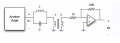The Electrician already showed me how to solve for transfer function between any node, input/output impedance by considering non-ideal transformer. By using non-ideal transformer, we can get the admittance matrix for the transformer and then the admittance matrix of the whole circuit.

After getting the admittance matrix for the whole circuit, we can find transfer function between any node, input/output impedance for the case non-ideal transformer.
Finally taking the limits of transfer function between any node, input/output impedance above to get the results for the circuit with ideal transformer.

The ideal transformer doesn't have an admittance matrix. However, by adding R1 and R2 we can write the admittance matrix for the transformer.
Then we setup the admittance matrix for the whole circuit. Finally taking the limit (R1--> 0 and R2 --> ∞) to get the admittance matrix for the whole circuit with ideal transformer.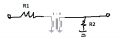With this non-ideal transformer, I get the admittance matrix: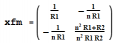If I designate nodes as below, I get the total admittance matrix for the whole circuit with non-ideal transformer.

Note: A is the gain of the amplifier.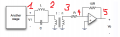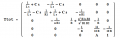Then for all calculation after that such as transfer function between any node, input/output impedance with non-ideal transformer are calculated from this total admittance matrix.

The transfer function between any node, input/output impedance with ideal transformer can be obtained by taking the limit of transfer function between any node, input/output impedance with non-ideal transformer with R1--> 0 and R2 --> ∞.

This is how to solve transfer function, input/output impedance using admittance matrix for the case with ideal transformer.

However, I believe that the thesis by Jacob Shekel describes the method to get the admittance matrix for the whole circuit containing the ideal transformer without having to using non-ideal model and then take the limits as above.

Also, are you familiar with the "T" network transformer model?
I know about T model for BJT but I haven't heard about the "T" network transformer model yet.

Last edited:

#### MrAl

Joined Jun 17, 2014
10,608
Hi MrAl,

Yes.
For example with this circuit below.

View attachment 147038

The Electrician already showed me how to solve for transfer function between any node, input/output impedance by considering non-ideal transformer. By using non-ideal transformer, we can get the admittance matrix for the transformer and then the admittance matrix of the whole circuit.

After getting the admittance matrix for the whole circuit, we can find transfer function between any node, input/output impedance for the case non-ideal transformer.
Finally taking the limits of transfer function between any node, input/output impedance above to get the results for the circuit with ideal transformer.

The ideal transformer doesn't have an admittance matrix. However, by adding R1 and R2 we can write the admittance matrix for the transformer.
Then we setup the admittance matrix for the whole circuit. Finally taking the limit (R1--> 0 and R2 --> ∞) to get the admittance matrix for the whole circuit with ideal transformer.
View attachment 147039

With this non-ideal transformer, I get the admittance matrix:

View attachment 147040

If I designate nodes as below, I get the total admittance matrix for the whole circuit with non-ideal transformer.

Note: A is the gain of the amplifier.

View attachment 147042

View attachment 147041

Then for all calculation after that such as transfer function between any node, input/output impedance with non-ideal transformer are calculated from this total admittance matrix.

The transfer function between any node, input/output impedance with ideal transformer can be obtained by taking the limit of transfer function between any node, input/output impedance with non-ideal transformer with R1--> 0 and R2 --> ∞.

This is how to solve transfer function, input/output impedance using admittance matrix for the case with ideal transformer.

However, I believe that the thesis by Jacob Shekel describes the method to get the admittance matrix for the whole circuit containing the ideal transformer without having to using non-ideal model and then take the limits as above.

I know about T model for BJT but I haven't heard about the "T" network transformer model yet.

Hello again,

I think i understand what you are looking for now as you seem to have explained it pretty good. You want to be able to write the equations without having to resort to a too indirect method which introduces other complications.

Well i think this is good, and also exploring the possibilities is also interesting. Using two resistors is interesting too.
Something else a little interesting too would be to 'map' R2 to R1, or map R1 to R2.
Make R2=1/R1, then just take the limit as R1 goes to zero, or alternately make R1=1/R2, and take the limit as R2 goes to infinity. Did you try that yet? Note this isnt what you were looking for, but just another little exploration of the technique.

The "T" equivalent model of the transformer is just a three inductor model of the transformer where the three inductors are arranged into a shape that looks like a "T". Each inductor represents a certain parameter of the real transformer, however there is no electrical isolation.
You can look this up or i can provide details on this model. The lower 'leg' of the model is the mutual inductance.
The nice thing about this model is you can make it ideal or non ideal by just changing the 'k' factor which i am sure you know about (for transformers of course). The drawback is it still introduces a host of sundry elements that may not be really needed in an ideal transformer.

There's one more model that could work here though, and that is (for lack of a better name) the bilateral current/voltage model. This might be called simply the "DC" model of a transformer. I'll have to look up this model as i cant remember offhand the arrangement it uses to accomplish the task of acting like a transformer while not introducing other little components here and there. It involves using controlled sources, and i have a feeling that paper uses the same idea except that was so long ago it may not have been apparent that what was being done, or perhpas that is the actual origin of this model, which would be interesting too.

I'll see if i can find this model, and i assume that you know how to write the equations using controlled sources too ok?
In the mean time, try the R1 and R2 'mapping' and see which one works, that would be a little interesting too.

Just to note, i dont have a problem with using R1, R2, or both R1 and R2 really, but i do see your point. Using R1 introduces one more node for one thing, so if we had 10 transformers in the circuit we'd be adding 10 more nodes. Most of the circuits we do on these forums are usually simple enough though so it doesnt matter that much if we add one more node.

Oh yeah another point is isolation. None of the models probably provide that directly. I meant to address this issue in the past but never actually got to it yet. For example the DC model will pass DC just as it would AC, unlike a real transformer with two real windings. Lucky there are ways around that.

#### MrAl

Joined Jun 17, 2014
10,608
Hello again,

Ok here is the DC model. Since it uses just controlled sources the entries should be just gains or something.

Note the polarities are very important here, and the 'gain' of each source must be the same. In the example, we have a gain of 2 for both elements, and the input is 2v and the output is 4v as expected with a gain of 2. Not shown is if the 'output' is excited the input will assume the inverse gain, which would be 1/2, so 2v on the 'output' would produce 1v on the 'input.
Note i have to put 'input' and 'output' in quotes because since this is a bilateral element they both act the same except for the transfer gain.

Try writing the equation for a simple circuit using a transformer and see if this helps. It should.
Components could then later be added to make this non ideal if desired.

I'm going to play with this a little more myself too.

#### anhnha

Joined Apr 19, 2012
902
Hi MrAl,

I think i understand what you are looking for now as you seem to have explained it pretty good. You want to be able to write the equations without having to resort to a too indirect method which introduces other complications.
Yes. If you look at the section 3.7 How to Write the Y Matrix of a Given Network (page 30) in the thesis I attached in the first post, the author gives an example explaining how to write the Y matrix in figure 9 with a voltage regulator.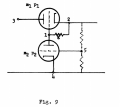The final admittance matrix doesn't have to use any variable R1 (upper resistor) and R2 (low resistor) of the voltage divider. It only uses feedback factor k with a voltage constraint:

(V5 - V4) = k(V2-V4)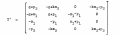If we use normal approach, we can model R1, R2 of the non-ideal voltage divider as below. Then set up admittance matrix for the whole circuit, calculate all interested things such as transfer function, input/output impedance. And finally take the limit of that results (transfer functions, impedance, etc) with R--> ∞ (mimic an ideal voltage divider that draws no current) and done. This method will maybe cause more node and have to take limits which I don't like much.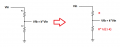As you can see the admittance matrix Y' above doesn't need to use R and doesn't have to take the limit as it only uses ideal feedback factor k.
The total admittance matrix is written by first writing admittance matrix of the circuit without the ideal voltage divider. Then we modify the obtained admittance matrix by adding a voltage constraint (V5 - V4) = k(V2-V4) with k < 1 to get the final admittance matrix of the whole circuit.

So I am looking for a similar approach and use it for the case of ideal transformer by something like this:

+ First write the admittance matrix for the circuit without the ideal transformer
+ Next modify the obtained admittance matrix with voltage and current constraints of the ideal transformer (Vout = n*Vin and Iin = n*Iout)

So the total admittance matrix doesn't include any parasitic parameters. It only includes turn ration n. So we can calculate all interested transfer functions directly from this matrix without having to taking any limits.

Well i think this is good, and also exploring the possibilities is also interesting. Using two resistors is interesting too.
Something else a little interesting too would be to 'map' R2 to R1, or map R1 to R2.
Make R2=1/R1, then just take the limit as R1 goes to zero, or alternately make R1=1/R2, and take the limit as R2 goes to infinity. Did you try that yet? Note this isnt what you were looking for, but just another little exploration of the technique.
That is an interesting idea as we can reduce the number of variables while taking limits. For the example in the first post, I have to take 3 limits A--> ∞, R1--> 0 and R2--> ∞. But with the idea, only two limits are needed.
I haven't tried it yet but I am sure it works and simplify the calculation.

I'll see if i can find this model, and i assume that you know how to write the equations using controlled sources too ok?
In the mean time, try the R1 and R2 'mapping' and see which one works, that would be a little interesting too.
Yes. I've just tried that and as expected it works well. This is one improvement but what I am looking for is not having to taking any limits for the transformer.

Just to note, i dont have a problem with using R1, R2, or both R1 and R2 really, but i do see your point. Using R1 introduces one more node for one thing, so if we had 10 transformers in the circuit we'd be adding 10 more nodes. Most of the circuits we do on these forums are usually simple enough though so it doesnt matter that much if we add one more node.
Exactly, also having to taking a lot of limits is not easy too. With multivariables limit, the order of taking limit also important and it may cause different result in some cases. For example, with 3 variables A--> ∞, R1--> 0 and R2--> ∞, there are 6 ways to limit and sometimes I get lost not knowing which one is correct.

With DC transformer model, I think I get what you meant. I have used it in modeling small signal DC-DC converters.

#### MrAl

Joined Jun 17, 2014
10,608
Hello again,

Ok well using his method is going to take some reading on my part, maybe a lot of readingBut one thing stikes me right away, and that is that the 'triode' example, doesnt that include plate conductance or something? That would be analogous to our resistors, but only one resistor instead of two.

But also did you try writing the equaions with the DC transformer model? That simplifies things a lot too. There are no resistors, but the output current (that controls the input current) may have to be developed from the circuit to the right of the transformer.
What i did was i used ONE resistor on the output, and that allowed me to calculate just about anything i wanted to do. That resistor is a current sense resistor to make it easier, and that might be analogous to the plate conductance used in the triode example.

I'll have to do more reading also and see what comes of it. That paper looks old old and too bad it uses a vacuum tube as an example.

#### anhnha

Joined Apr 19, 2012
902
Hi MrAl,

Ok well using his method is going to take some reading on my part, maybe a lot of reading
I am sorry about this but I thought you only need to read section 3.6 and 3.7 alone because it doesn't seem to related much to previous one.

But one thing stikes me right away, and that is that the 'triode' example, doesnt that include plate conductanceorsomething?That would be analogous to our resistors, but only one resistor instead of two.
In the picture above m is the mutual grid-to-plate transconductance, and p the internal plate conductance.

But also did you try writing the equaions with the DC transformer model? That simplifies things a lot too. There are no resistors, but the output current (that controls the input current) may have to be developed from the circuit to the right of the transformer.
What i did was i used ONE resistor on the output, and that allowed me to calculate just about anything i wanted to do. That resistor is a current sense resistor to make it easier, and that might be analogous to the plate conductance used in the triode example.
Actually I don't know how to start with this. What should I do, if possible could you provide the model and tell me more detail what to do?

I'll have to do more reading also and see what comes of it. That paper looks old old and too bad it uses a vacuum tube as an example.
Yes, I had to review vacuum tube. However, if you don't like it, we can replace it by BJT as the issue is the ideal voltage divider not the tube.

#### MrAl

Joined Jun 17, 2014
10,608
Hello again,

Wow i cant believe that happened again, either i never uploaded the circuit or it did not get accepted for some reason, so i will upload it again now.

Also, i am not entire sure i know what you mean when you say you want an idea voltage divider.
Can you show your matrix with just an ideal voltage divider and input series resistor and output load resistor? (no triode)

Here is the DC transformer model. See what you think.
Notice 2v in and 4v out, because the ratio is 1:2 and so both gains are 2.
Now an impedance on the input will reflect to the output as a regular transformer would, and an impedance on the output would reflect back to the input as a transformer would.
The circuit shown is an 'ideal' transformer but we could add stuff to make it non ideal if needed. Of course it works with DC as well as AC.

Also, i dont think i made it clear what i was saying about the triode vs the transformer. If the triode example uses a plate conductance, then why cant we use a conductance along with the transformer. That makes it easy right? There's no taking of limits if the conductance is always there. Perhaps your rendition of the ideal voltage divider in a simple resistive circuit will shed some more light on this.
I also read now that gm=u/rp where rp is the plate resistance so gm=u*gp with gp plate conductance. So that's not ideal is it?

I almost forgot to mention...
Another technique is impedance reflection. That is where we reflect the impedance of say the output circuit to the input circuit, thus eliminating the ideal transformer altogether. This results in a network that is just a regular network with no transformer so you should be able to handle it as usual.
The impedances reflect either as a^2 or 1/a^2 depending on which way we are going, and 'a' here is the turns ratio.
A quick example is a 1:2 ratio with 1 Ohm on the output terminals.
With 1 volt on the input we get 2v on the output so we get 2 amps on the output so we have 4 watts on the output so we have 4 watts on the input, and with 1v that means 4 amps. 1v/4a means R=0.25 ohms, so going forward 0.25*a^2=1 and going backwards 1/a^2=0.25, and of course a=2 here which is the turns ratio primary to secondary.

Last edited:

#### anhnha

Joined Apr 19, 2012
902
Hi MrAl,

I tried the case of a simple circuit including ideal voltage divider as you suggested below but then I realized that there is a problem with that circuit. The admittance for that circuit doesn't include feedback factor k for the ideal voltage divider.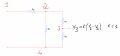For that reason, let's start with the following circuit that is a little bit more complex but simple enough to show the theory.
Also for the voltage divider, I map the lower resistor to the upper resistor so we can reduce one variable.
For the ideal voltage divider, it should draw no current - meaning that R --> ∞. However, below I will take the approach given in the thesis to write the admittance matrix for this circuit that only have feedback factor k and no need to include R any more.First, let's start with the definition of indefinite admittance matrix from this book. I believe you know this very well but I'd like to metion it for completeness.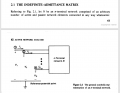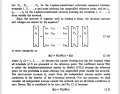Now let's start writing the indefinite admittance matrix for a MOS transitor. Note that all currents are defined flowing into its terminal.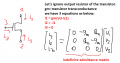Now come back to the circuit with voltage divider above. Our goal is to find the indefinite admittance for that circuit with ideal voltage voltage divider - meaning that the admittance matrix only includes feedback factor k and not include resistor R.Here is the approach from the thesis (if I understand it correctly):

First, write the indefinite admittance matrix for the circuit without the ideal-voltage divider.
By inspection, we can write the admittance matrix as below: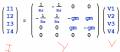Next, let's adding the voltage constraint by the ideal-voltage divider into the matrix.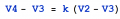Now let's definite V1, V2, V3 and V4 with the voltage constraint above (eliminating V4 from computation):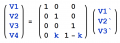Continue in the next post...

#### Attachments

Last edited:
•Jony130

#### anhnha

Joined Apr 19, 2012
902
Continue...
Now replace the the new defintion into the equation I = Y.V above we have: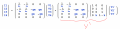Or: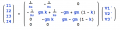We see that I4 can also be removed so finally we have: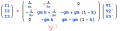Y' is the final indefinite admittance matrix for the circuit including ideal-voltage divider that we want to find. V1, V2,V3 is equal to V1, V2, V3 as we redefined above.

This is the approach from the thesis. The thesis gives a systemmatic way to do that by two steps.

Step 1: find the admittance without the voltage constraint
Step 2: base on the voltage constraint, we can find find a matrix including the constraint.
--> The admittance matrix for the circuit from 2 matrices above.

I see that we can draw small signal model and add the constraint from the begining and then find the matrix. However, I would like to follow the systemmatic method as the thesis.

Also, I am wondering how to apply that to the ideal transformer.

Last edited:
•Jony130

#### anhnha

Joined Apr 19, 2012
902
Actually, my derivation in post #11 is a little bit different from the thesis.
Here is what is done as in the thesis:
First, we have the admittance matrix without the voltage divider: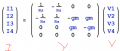Next, using the voltage constraint we redefine: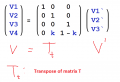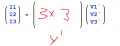The indefinite admittance matrix Y` is calculated as below. The proof is given in section 3.6 of the thesis.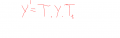Last edited:
•Jony130

#### MrAl

Joined Jun 17, 2014
10,608
Hi,

Well doing this with a transformer is going to take some thought. For one thing, the MOS transistor is not bilateral in the same way a transformer is. Do we have any guarantee that this does in fact work with a transformer?

I cant remember if i solved the problem of the DC transformer with one output resistor. That is, getting rid of that one resistor right away.
In many circuits though you dont even have to take a limit. The extra resistor disappears just by setting it to zero in all solution equations.
For example, a solution might be:
Vout=Vin*(R1+R3)/(R1+R2+R3)

and setting R3=0 we easily get:
Vout=Vin*R1/(R1+R2)

so it is not as hard as when we actually have to take a limit. Of course i cant say for sure if this simplification is possible in every possible circuit we can ever encounter.

#### anhnha

Joined Apr 19, 2012
902
Hi MrAl,
I have just tried the Shekel theory with the transformer circuit in my post #3 and it works.
I only need to use only one constraints - voltage constraint for the transformer as the theory assumes that no loss so if we assume voltage constraint then the current constraint is just a a result as proof in section 3.6 of the thesis.
I am doing the DC transformer model so I'll get back when I have some result.

#### anhnha

Joined Apr 19, 2012
902
Hello again,

Ok here is the DC model. Since it uses just controlled sources the entries should be just gains or something.

Note the polarities are very important here, and the 'gain' of each source must be the same. In the example, we have a gain of 2 for both elements, and the input is 2v and the output is 4v as expected with a gain of 2. Not shown is if the 'output' is excited the input will assume the inverse gain, which would be 1/2, so 2v on the 'output' would produce 1v on the 'input.
Note i have to put 'input' and 'output' in quotes because since this is a bilateral element they both act the same except for the transfer gain.

Try writing the equation for a simple circuit using a transformer and see if this helps. It should.
Components could then later be added to make this non ideal if desired.

I'm going to play with this a little more myself too.
Hi MrAl,

To make it general, let's assume the transformer return ratio is n.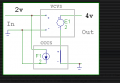Now I am not sure what is the equation you meant to write.

Vout = n*Vin
Iin = n*Iout

#### MrAl

Joined Jun 17, 2014
10,608
Hello,

Well usually 'a' is used to show the 'gain'.
The basic relationships are:
Vout=a*Vin
Iout=Iin/a
Rin=Rout/a^2
Rout=a^2*Rin
where Rin and Rout are really Zin and Zout respectively.
Thus for example:
Iout=(a*Vin)/Rout=Vin/(a^2*Rin)

Once this is placed into a circuit, the Rin and Rout have significance.
I was thinking this might help you write the equations you want to write.

It is after all a transformer so once it is placed into a circuit it provides equations that are what they should be, so you just have to figure out how to turn it into the matrix you want. Maybe you could try doing the same thing with this model that you did with the Mos transistor. You could even short out the bottom two leads to make it simpler (a typical application anyway). One difference will be that there will be no lead with zero current like there was with the Mos gate.

#### anhnha

Joined Apr 19, 2012
902
Hi MrAl,
Could you illustrate to use that with a simple circuit? I don't understand how to start with it. Four equations you wrote are the properties of a transformer. I am not sure if you meant to remodel it using these 4 equations?

#### MrAl

Joined Jun 17, 2014
10,608
Hi MrAl,
Could you illustrate to use that with a simple circuit? I don't understand how to start with it. Four equations you wrote are the properties of a transformer. I am not sure if you meant to remodel it using these 4 equations?
Hi,

As funny as this will sound, i am not even sure that i understand what it is that you dont understand.
Why would you suggest that i was to "remodel' the transformer just because i wrote four equations for the transformer?

I will put it in a simple circuit and post results here so that might help you get an idea what this is leading to.
Give me a little while to prepare everything. I'll make another post so it shows up again in your 'alerts' list.

The basic idea though is simply that if we want to write any equations for anything we have to have a circuit to start with, and if we are concentrating on one single type of part then we need a model for that part or else we have no place to start. That is of course unless someone hands us a set of equations, which isnt happening here.

#### MrAl

Joined Jun 17, 2014
10,608
Hi,

As funny as this will sound, i am not even sure that i understand what it is that you dont understand.
Why would you suggest that i was to "remodel' the transformer just because i wrote four equations for the transformer?

I will put it in a simple circuit and post results here so that might help you get an idea what this is leading to.
Give me a little while to prepare everything. I'll make another post so it shows up again in your 'alerts' list.

The basic idea though is simply that if we want to write any equations for anything we have to have a circuit to start with, and if we are concentrating on one single type of part then we need a model for that part or else we have no place to start. That is of course unless someone hands us a set of equations, which isnt happening here.
Hello again,

Did you say you did it with the transformer already then? I might have missed that post.

Here is a writeup for the DC transformer.
Note we start with a constant current source instead of a CCCS and then later change it to a CCCS source once we get the matrix.

This is a solution for voltages, so i did not show the impedance solution yet. The impedance transformation solution eliminates the transformer completely.

Small note:
The lower case v1, v2, are voltages, the upper case V1 is a vector.
Also, once R3=0 there should be a way to reduce the matrix by 1 row and column and vector by 1 row before doing the solution.

#### anhnha

Joined Apr 19, 2012
902
Hi MrAl,

Sorry for the late...
It is an interesting approach. I think your method works well. In your model, is R3 added just to model the transformer?
I see that setting R3 = 0 is easy too.
Also is there an easy way to write the matrix just by inspection?
In the matrix, you replaced I1 by (V2-V3)*A/R3. How to determine of the sign here? Why not (V3-V2)*A/R3?

Last edited: